Ch 5. Rigid Body General Motion Multimedia Engineering Dynamics Fixed Axis Rotation Plane Motion Velocities Zero Velocity Point Plane Motion Accelerations Multiple Gears Rot. Coord. Velocities Rot. Coord. Acceleration
 Chapter - Particle - 1. General Motion 2. Force & Accel. 3. Energy 4. Momentum - Rigid Body - 5. General Motion 6. Force & Accel. 7. Energy 8. Momentum 9. 3-D Motion 10. Vibrations Appendix Basic Math Units Basic Equations Sections Search eBooks Dynamics Fluids Math Mechanics Statics Thermodynamics Author(s): Kurt Gramoll ©Kurt GramollDYNAMICS - THEORYExample of rotating rigid bodies with
moving point on one body

Previously, multiple rigid bodies that were connected with pin joints were analyzed. This section considers the same rigid body motion, but now a point on one of the rigid bodies is allowed to moved and two coordinate systems are used to model the motion. A good example of this type of motion would be two links connected with a sliding connection, as shown in the diagram.

To analyze a rotating rigid body with a moving point we need to start with the basic relative position vector equation and take a time derivative. To help with modeling complex systems, two coordinate systems are used: X-Y is fixed and x-y rotates in the X-Y system. Note, x-y system is not fixed to the body.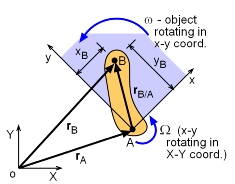Position vectors

rB = rA + rB/A

The first time derivative gives,

d(rB)/dt = d(rA + rB/A)/dt

vB = vA + d(rB/A)/dt

A simple derivative for rB/A is not correct since the coordinate system, x-y, also is changing which must be accounted for when taking a derivative.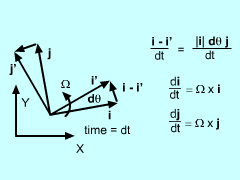Time Derivative of i and j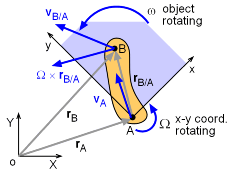Velocity vectors

Using xB and yB as the scalar components of rB/A gives,

vB = vA + d(xAi + yBj)/dt

It is important to note that di/dt ≠ 0 and dj/dt ≠ 0 since i and j can rotate. Using the chain rule, this can be expanded as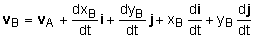The unit vectors, i and j, can rotate so their time derivatives are di/dt = Ω × i and dj/dt = Ω × j. Also, note that dxB/dt i and dyB/dt j is simply the relative velocity of B with respect to A. This gives

vB = vA + (vB/A)rel + xBΩj + yBΩi

The last two terms can be converted to a cross product to give

 vB = vA + Ω × rB/A + (vB/A)rel

This is the classic form of the relative motion equation using rotating axes. The key term is Ω × rB/A which relates the x-y coordinate system with the X-Y coordinate system.

Coordinate Transformation

Since two coordinates are involved with most rotating coordinate system problems, they need to be related. In other words, one coordinate system needs to be mapped into the other, and vce versa. Relating two coordinate system is a common task in most all engineering fields, not just dynamics.Velocity vectors

The easiest way to relate coordinate systems is to descripbe one system in the other, just like is done for a point or vector. For example, the i unit-vector i can be described in the coordinate system XY as

i = cosθ I + sinθ J

likewise, the j unit-vector is

j = -sinθ I + cosθ

These relationships can be inverted to describe I and J using i and j unit-vectors

I =  cosθ I - sinθ J
J = sinθ I  + cosθ J

These transformation equations are commonly written in matrix form, and called the 2D coordinate transformation matrix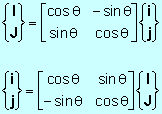They can be used for any two coordinate systems where the xy-system is rotated an angle θ relative to the XY-system.

Practice Homework and Test problems now available in the 'Eng Dynamics' mobile app
Includes over 400 problems with complete detailed solutions.
Available now at the Google Play Store and Apple App Store.